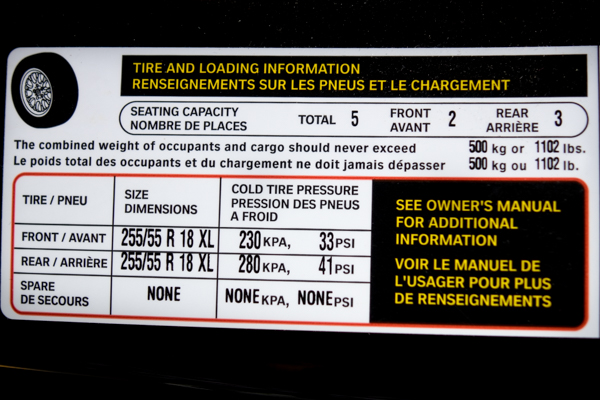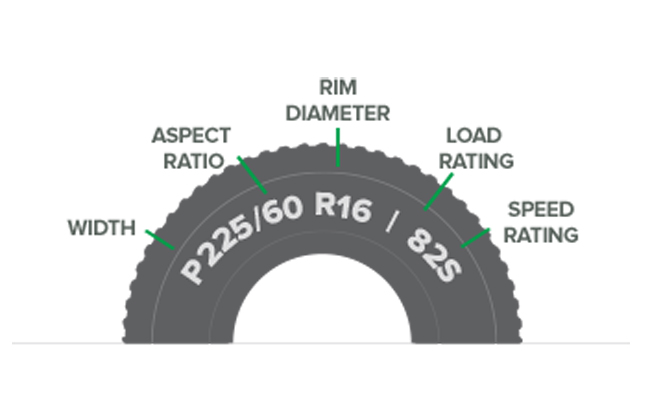### Where can I find my tire size?

The tire size for your vehicle and wheels can be found in two places:

2. Inside frame of the driver side door

Tire Size Example: 255/55 R18 XL### What does my tire size mean?

Width

• The first three numbers of a tire size measures the width in millimeters from sidewall to sidewall.
• If the tire size is 255/60 R16, the width of the tire is 255 millimeters.

Aspect Ratio

• The two digit number after the slash mark is the aspect ratio which measures the tire's cross-section to it's width.
• If the tire size is 255/60 R16, the 60 means that the height is 60% of the tire's width. The bigger the aspect ratio, the bigger the tire's sidewall will be.

Construction

• In between the Aspect Ratio and Rim Diameter, is the construction of the tire.
• If the tire size is 255/60 R16, the "R" stands for Radial, which means the layers of the tire run in straight lines across the tire.
• If there is a "B" instead of an "R" listed on your tires, the "B" stands for Bias construction which means the layers run diagonally across the tire.

Wheel Size (Rim Diameter)

• Located after Construction is the wheel size, which tells us the size of the wheel/rim that the tire is intended to fit and is measured from one end of the wheel to the other.
• If the tire size is 255/60 R16, the 16 means that the wheel is 16" in diameter.

• To support the weight of the vehicle, each tire is assigned a designated number that coincides with the maximum weight that the tire can support when it has been inflated.
• If your tire size is 255/60 R16/82S, the load rating/index would be 82 meaning the load in lbs would be 1,047lbs.
• A high tire load index number means the tire can hold a a greater load carrying capacity.
• If you purchase a tire with a lower load index than what is recommended for your vehicle, the tire will not be able to carry the weight of the vehicle.